# PyTorch for Recommenders 101

Recommenders, generally associated with e-commerce, sift though a huge inventory of available items to find and recommend ones that a user will like. Different from search, recommenders rely on historical data to tease out user preference. How does a recommender accomplish this? In this post we explore building simple recommendation systems in PyTorch using the Movielens 100K data, which has 100,000 ratings (1-5) that 943 users provided on 1682 movies.

## Matrix Factorization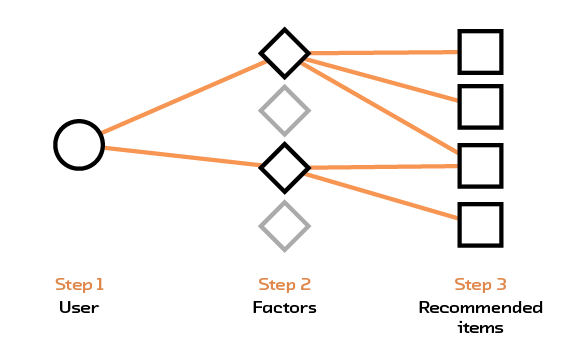We first build a traditional recommendation system based on matrix factorization. The input data is an interaction matrix where each row represents a user and each column represents an item. The rating assigned by a user for a particular item is found in the corresponding row and column of the interaction matrix. This matrix is generally large but sparse; there are many items and users but a single user would only have interacted with a small subset of items. Matrix factorization decomposes this larger matrix into two smaller matrices - the first one maps users into a set of factors and the second maps items into the same set of factors. Multiplying these two smaller matrices together gives an approximation to the original matrix, with values for empty elements inferred. To predict a rating for a user-item pair, we simply multiply the row representing the user from the first matrix with the column representing the item from the second matrix.

In PyTorch we can implement a version of matrix factorization by using the embedding layer to “map” users into a set of factors. The number of factors determine the size of the embedding vector. Similarly we map items into their own embedding layer. Both user and item embeddings have the same size. To predict a user-item rating, we multiply the user embeddings with item embeddings and sum to obtain one number. The following code draws from Ethan Rosenthal’s work on matrix factorization in PyTorch.

import torch
from torch.autograd import Variable

class MatrixFactorization(torch.nn.Module):
def __init__(self, n_users, n_items, n_factors=20):
super().__init__()
# create user embeddings
self.user_factors = torch.nn.Embedding(n_users, n_factors,
sparse=True)
# create item embeddings
self.item_factors = torch.nn.Embedding(n_items, n_factors,
sparse=True)

def forward(self, user, item):
# matrix multiplication
return (self.user_factors(user)*self.item_factors(item)).sum(1)

def predict(self, user, item):
return self.forward(user, item)



To fit the matrix factorization model we need to pick a loss function and an optimizer. In this example we use the average squared distance between the prediction and the actual value as a loss function, this is known as mean-squared error. We then try to minimize this loss by using stochastic gradient descent. The code below shows how the model is fitted in four steps: i) pass in a user-item pair, ii) forward pass to compute the predicted rating, iii) compute the loss, and iv) backpropagate to compute gradients and update the weights.

model = MatrixFactorization(n_users, n_items, n_factors=20)
loss_fn = torch.nn.MSELoss()
optimizer = torch.optim.SGD(model.parameters(),
lr=1e-6)

for user, item in zip(users, items):
# get user, item and rating data
rating = Variable(torch.FloatTensor([ratings[user, item]]))
user = Variable(torch.LongTensor([int(user)]))
item = Variable(torch.LongTensor([int(item)]))

# predict
prediction = model(user, item)
loss = loss_fn(prediction, rating)

# backpropagate
loss.backward()

# update weights
optimizer.step()


We train this model on the Movielens dataset with ratings scaled between [0, 1] to help with convergence. Applied on the test set, we obtain a root mean-squared error(RMSE) of 0.66. This means that on average, the difference between our prediction and the actual value is 0.66!

## Dense Feedforward Neural Network

Given the underwhelming performance of our matrix factorization model, we try a simple feedforward recommendation system instead. The input to this neural network is a pair of user and item represented by their IDs. Both user and item IDs first pass through an embedding layer. The output of the embedding layer, which are two embedding vectors, are then concatenated into one and passed into a linear network. The output of the linear network is one dimensional - representing the rating for the user-item pair. The model is fit the same way as the matrix factorization model and uses the standard PyTorch approach of forward passing, computing the loss, backpropagating and updating weights.

import torch
import torch.nn.functional as F
from torch.autograd import Variable
from torch import nn

class DenseNet(nn.Module):

def __init__(self, n_users, n_items, n_factors, H1, D_out):
"""
Simple Feedforward with Embeddings
"""
super().__init__()
# user and item embedding layers
self.user_factors = torch.nn.Embedding(n_users, n_factors,
sparse=True)
self.item_factors = torch.nn.Embedding(n_items, n_factors,
sparse=True)
# linear layers
self.linear1 = torch.nn.Linear(n_factors*2, H1)
self.linear2 = torch.nn.Linear(H1, D_out)

def forward(self, users, items):
users_embedding = self.user_factors(users)
items_embedding = self.item_factors(items)
# concatenate user and item embeddings to form input
x = torch.cat([users_embedding, items_embedding], 1)
h1_relu = F.relu(self.linear1(x))
output_scores = self.linear2(h1_relu)
return output_scores

def predict(self, users, items):
# return the score
output_scores = self.forward(users, items)
return output_scores



Once again, we train this model on the Movielens dataset with ratings scaled between [0, 1] to help with convergence. Applied on the test set, we obtain a root mean-squared error(RMSE) of 0.28, a substantial improvement!

## Sequence based Recommendation System

Finally we build a recommendation system that takes into account the sequence of item interactions. The heart of this is a Long Short-Term Memory (LSTM) cell, a variant of Recurrent Neural Networks (RNN) with faster convergence and better long term memory. The input to this system is a history of item interactions and their corresponding ratings. In the following example of an input, we show a sequence of item interaction of length 10 (arbitrarily chosen) and the corresponding rating. Elements in the first array correspond to items(movies), and elements in the second array correspond to ratings. We see that, for example, movie 209 has a rating of 4, and movie 32 has a rating of 5. Sequences shorter than 10 are padded with zeros.

In : training_data
Out:
(array([209,  32, 189, 242, 171, 111, 256,   5,  74, 102], dtype=int32),
array([4, 5, 3, 5, 5, 5, 4, 3, 1, 2], dtype=int32))


Items are passed through an embedding layer before going into the LSTM. The output of the LSTM is then fed into a linear layer with an output dimension of one. The LSTM has 2 hidden states, one for short term memory and one for long term. Both states need to be initialized.

PyTorch expects LSTM inputs to be a three dimensional tensor. The first dimension is the length of the sequence itself, the second represents the number of instances in a mini-batch, the third is the size of the actual input into the LSTM. Using our training data example with sequence of length 10 and embedding dimension of 20, input to the LSTM is a tensor of size 10x1x20 when we do not use mini batches. For a mini-batch size of 2, each forward pass will have two sequences, and the input to the LSTM needs to have a dimension of 10x2x20. LSTMs take variable input sequence lengths but for batch training purposes the input data is generally processed(with padding if necessary) to have a fixed length.

import torch
import torch.nn as nn
from torch.autograd import Variable

class LSTMRating(nn.Module):

def __init__(self, embedding_dim, hidden_dim, num_items, num_output):
super().__init__()
self.hidden_dim = hidden_dim
self.item_embeddings = nn.Embedding(num_items, embedding_dim)
self.lstm = nn.LSTM(embedding_dim, hidden_dim)
self.linear = nn.Linear(hidden_dim, num_output)
self.hidden = self.init_hidden()

def init_hidden(self):
# initialize both hidden layers
return (Variable(torch.zeros(1, 1, self.hidden_dim)),
Variable(torch.zeros(1, 1, self.hidden_dim)))

def forward(self, sequence):
embeddings = self.item_embeddings(sequence)
output, self.hidden = self.lstm(embeddings.view(len(sequence), 1, -1),
self.hidden)
rating_scores = self.linear(output.view(len(sequence), -1))
return rating_scores

def predict(self, sequence):
rating_scores = self.forward(sequence)
return rating_scores



Once the neural network is defined, we fit the training data using stochastic gradient descent with a mean squared error loss function.

embedding_dim = 64
hidden_dim = 128
n_output = 1

# add one to represent padding when there is not enough history
model = LSTMRating(embedding_dim, hidden_dim, n_items+1, n_output)
loss_fn = nn.MSELoss()
optimizer = optim.SGD(model.parameters(), lr=0.1)

for sequence, target_ratings in training_data:
# initialize hidden layers
model.hidden = model.init_hidden()
# convert sequence to PyTorch variables
sequence_var = Variable(torch.LongTensor(sequence.astype('int64')))
# forward pass
ratings_scores = model(sequence_var)
target_ratings_var = Variable(torch.FloatTensor(target_ratings.astype('float32')))
# compute loss
loss = loss_fn(ratings_scores, target_ratings_var)
# backpropagate
loss.backward()
# update weights
optimizer.step()



Similar to other models, we train the LSTM-based model on the Movielens dataset with ratings scaled between [0, 1] to help with convergence. Applied on the test set, we obtain a root mean-squared error(RMSE) of 0.43 - the LSTM model underperforms the dense feed forward network.

## Post-amble

The models discussed in this post are basic building blocks for a recommendation system in PyTorch. There are no bells and whistles and we did not attempt to fine tune any hyperparameters. Our first pass result suggests that the dense network performs best, followed by the LSTM network and finally the matrix factorization model. The root mean-squared error (RMSE) are 0.28, 0.43 and 0.66 respectively on the Movielens 100K dataset with ratings scaled between [0, 1]. We thought PyTorch was fun to use; models can be built and swapped out relatively easily. When we did encounter errors, most of them were triggered by incorrect data types.

For more on recommendations, please see our Semantic Recommendations report, where we focus on how machines can better understand content!

Older

## Latest posts

##### Sep 8, 2022 · post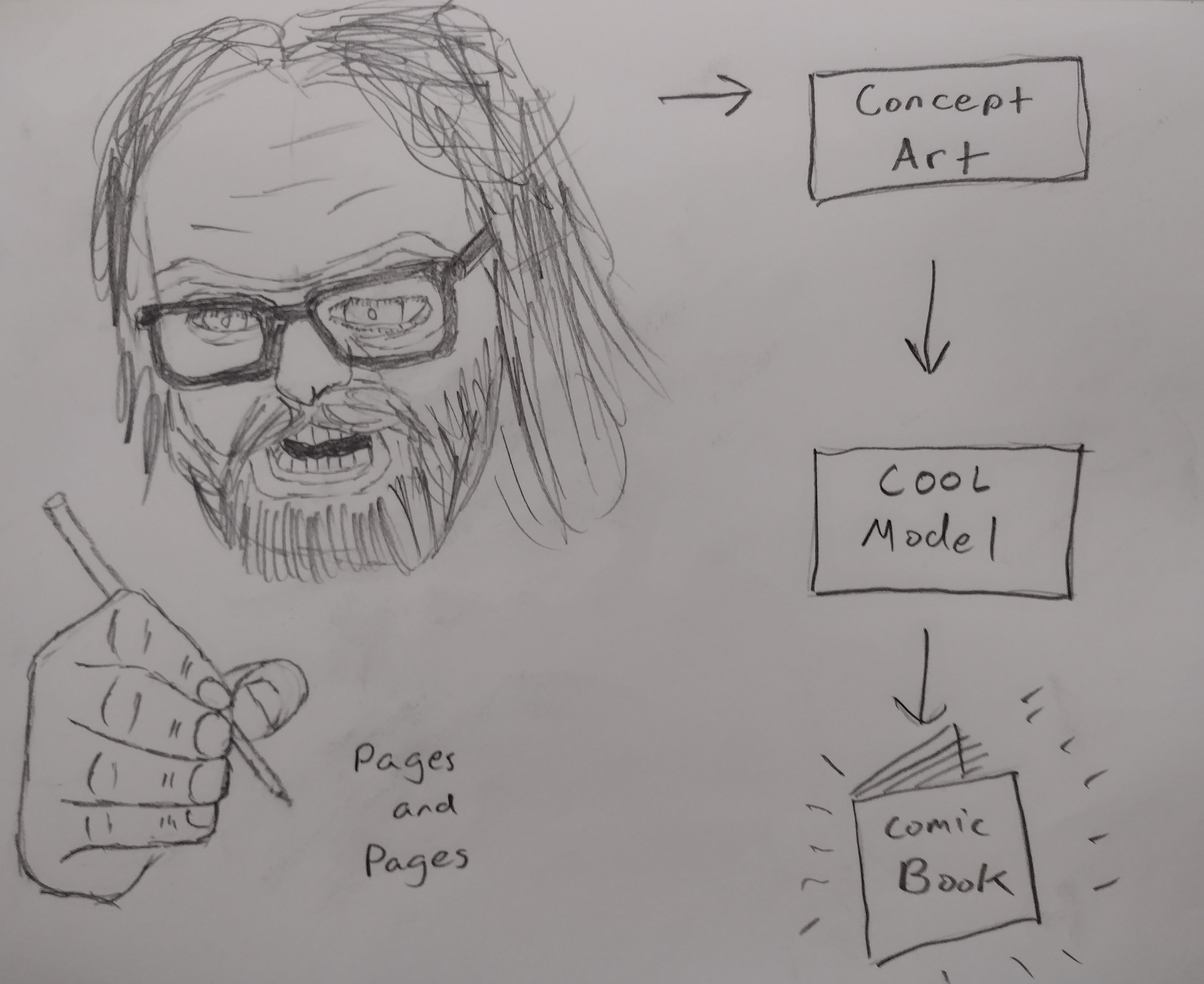by This post has a companion piece: Ethics Sheet for AI-assisted Comic Book Art Generation I want to make a comic book. Actually, I want to make tools for making comic books. See, the problem is, I can’t draw too good. I mean, I’m working on it. Check out these self portraits drawn 6 months apart: Left: “Sad Face”. February 2022. Right: “Eyyyy”. August 2022. But I have a long way to go until my illustrations would be considered professional quality, notwithstanding the time it would take me to develop the many other skills needed for making comic books.
##### Jul 29, 2022 · post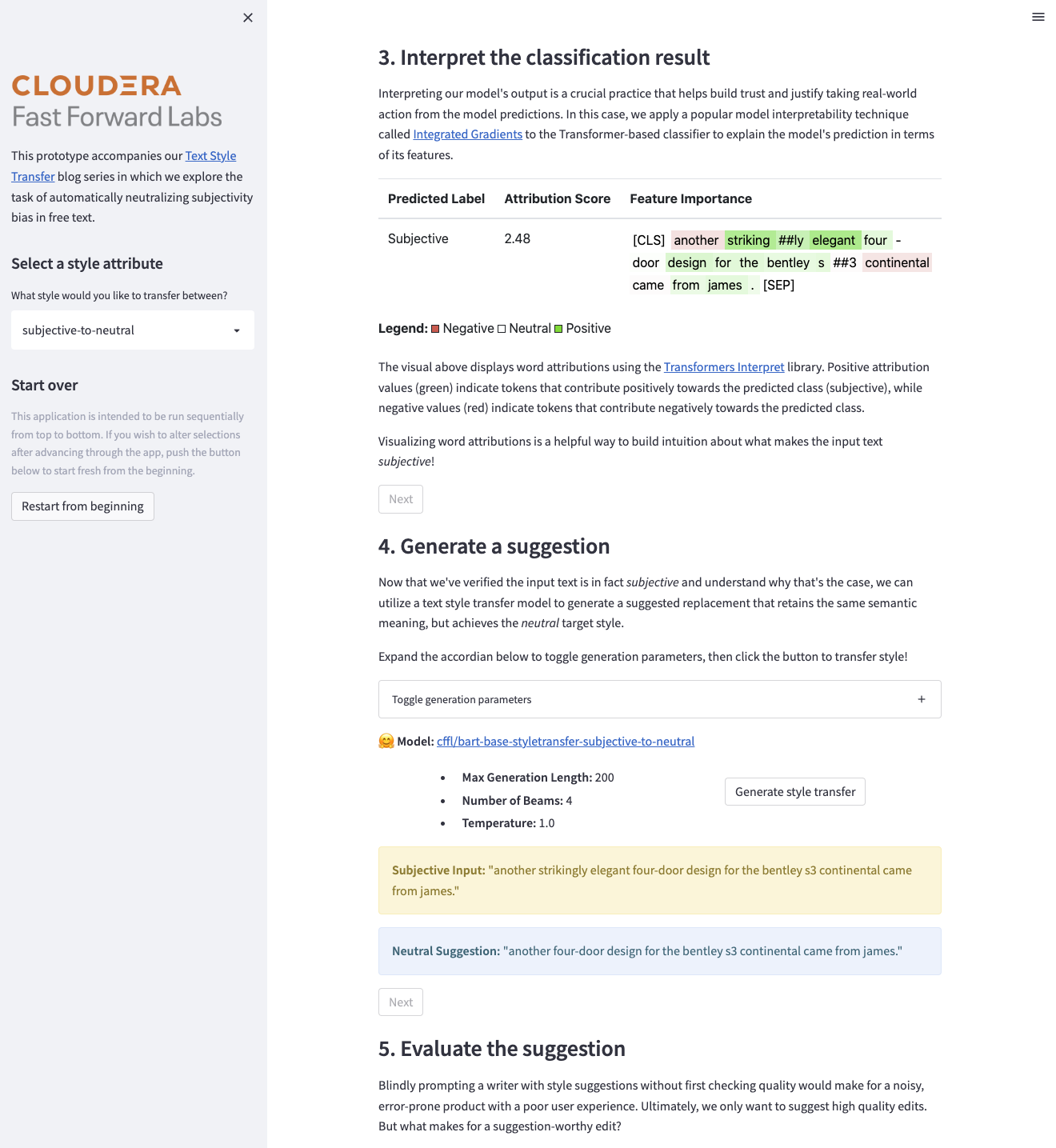by Blog Series This post serves as Part 4 of a four part blog series on the NLP task of Text Style Transfer. In this post, we expand our modeling efforts to a more challenging dataset and propose a set of custom evaluation metrics specific to our task. Part 1: An Introduction to Text Style Transfer Part 2: Neutralizing Subjectivity Bias with HuggingFace Transformers Part 3: Automated Metrics for Evaluating Text Style Transfer Part 4: Ethical Considerations When Designing an NLG System At last, we’ve made it to the final chapter of this blog series.
##### Jul 11, 2022 · post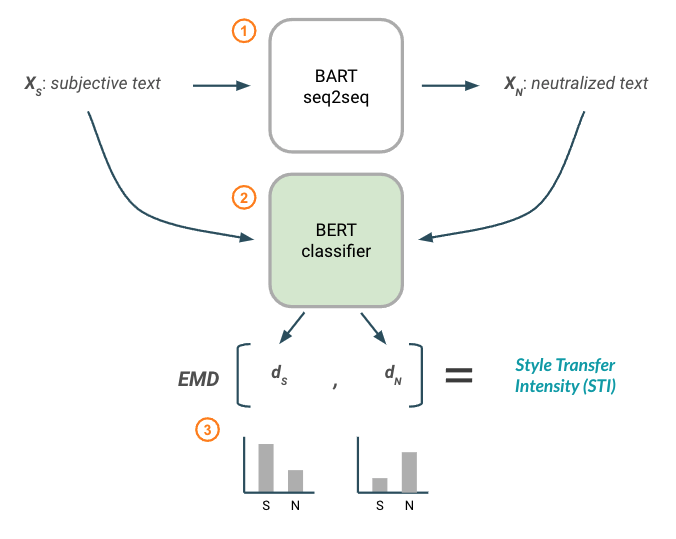by Andrew & Melanie · By Andrew Reed and Melanie Beck Blog Series This post serves as Part 3 of a four part blog series on the NLP task of Text Style Transfer. In this post, we expand our modeling efforts to a more challenging dataset and propose a set of custom evaluation metrics specific to our task. Part 1: An Introduction to Text Style Transfer Part 2: Neutralizing Subjectivity Bias with HuggingFace Transformers Part 3: Automated Metrics for Evaluating Text Style Transfer Part 4: Ethical Considerations When Designing an NLG System In our previous blog post, we took an in-depth look at how to neutralize subjectivity bias in text using HuggingFace transformers.
##### May 5, 2022 · post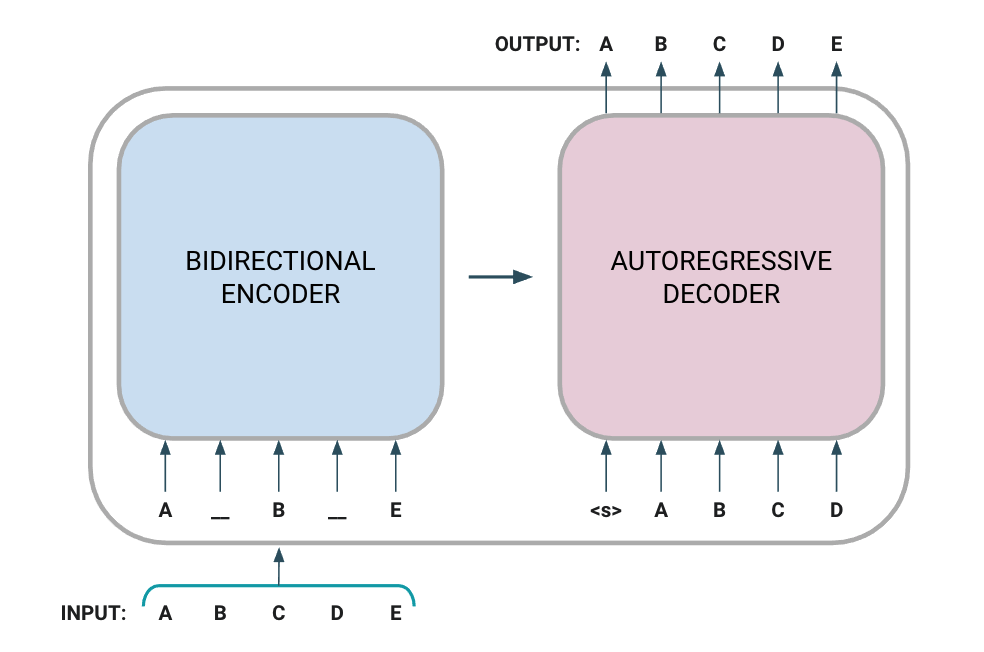by Blog Series This post serves as Part 2 of a four part blog series on the NLP task of Text Style Transfer. In this post, we expand our modeling efforts to a more challenging dataset and propose a set of custom evaluation metrics specific to our task. Part 1: An Introduction to Text Style Transfer Part 2: Neutralizing Subjectivity Bias with HuggingFace Transformers Part 3: Automated Metrics for Evaluating Text Style Transfer Part 4: Ethical Considerations When Designing an NLG System Subjective language is all around us – product advertisements, social marketing campaigns, personal opinion blogs, political propaganda, and news media, just to name a few examples.
##### Mar 22, 2022 · post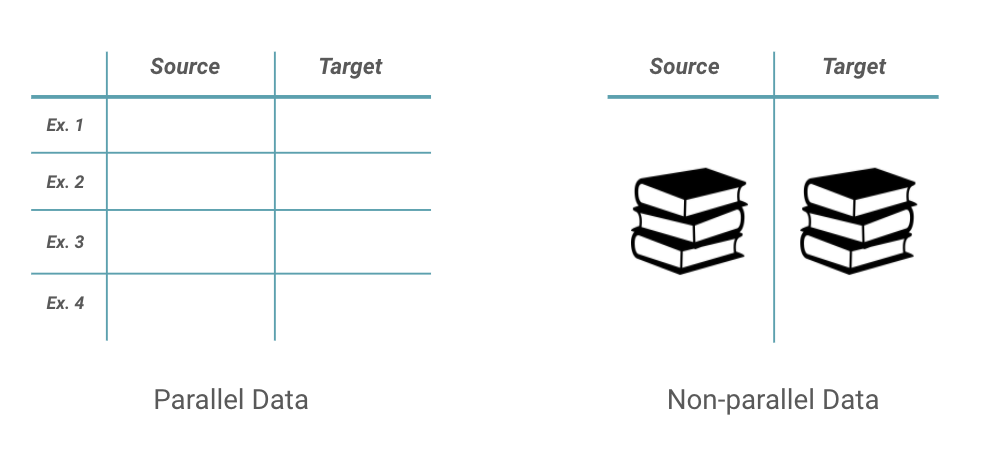by Blog Series This post serves as Part 1 of a four part blog series on the NLP task of Text Style Transfer. In this post, we expand our modeling efforts to a more challenging dataset and propose a set of custom evaluation metrics specific to our task. Part 1: An Introduction to Text Style Transfer Part 2: Neutralizing Subjectivity Bias with HuggingFace Transformers Part 3: Automated Metrics for Evaluating Text Style Transfer Part 4: Ethical Considerations When Designing an NLG System Today’s world of natural language processing (NLP) is driven by powerful transformer-based models that can automatically caption images, answer open-ended questions, engage in free dialog, and summarize long-form bodies of text – of course, with varying degrees of success.
##### Jan 31, 2022 · post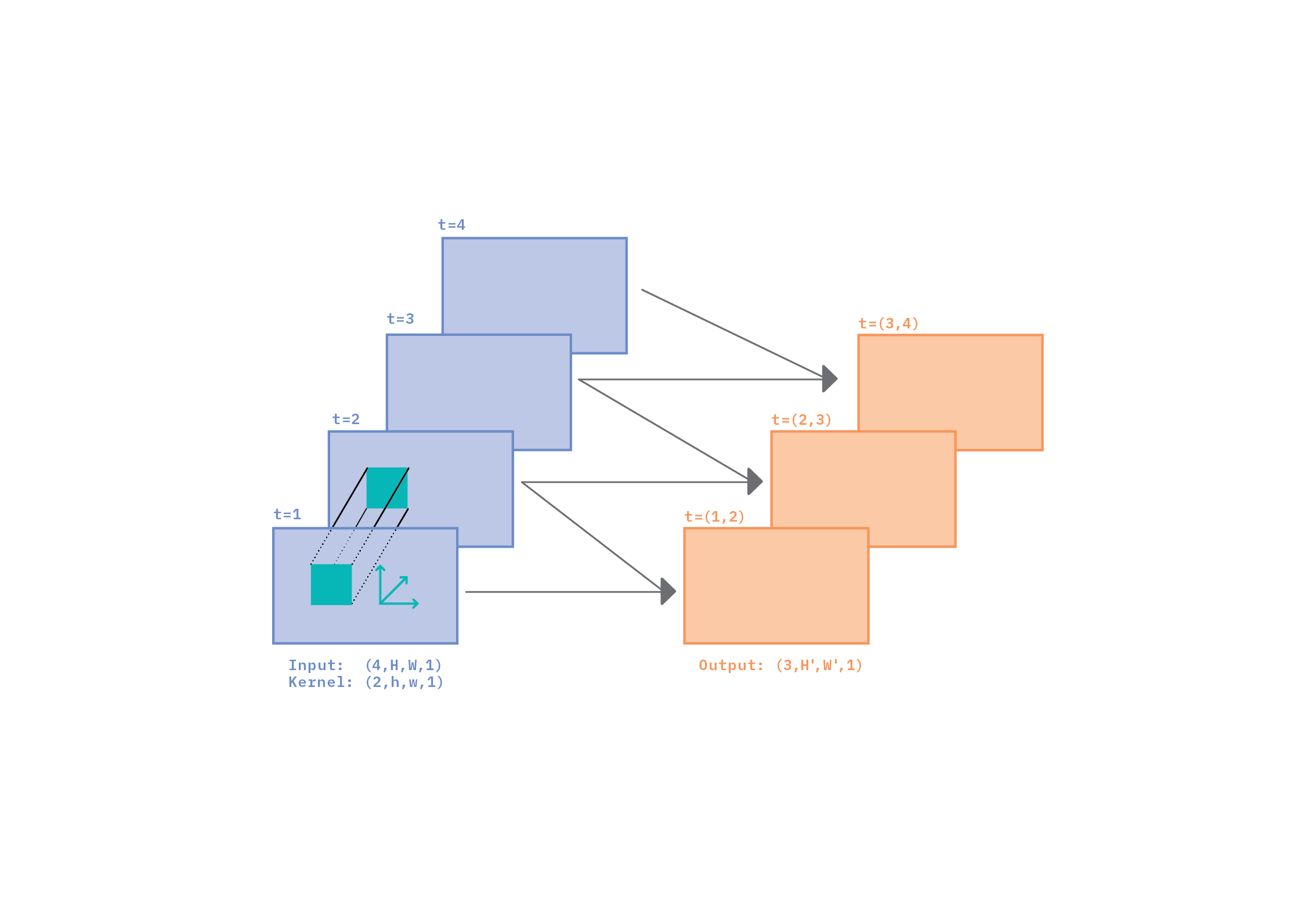by Video classification is perhaps the simplest and most fundamental of the tasks in the field of video understanding. In this blog post, we’ll take a deep dive into why and how convolutions work for video classification. Our goal is to help the reader develop an intuition about the relationship between space (the image part of video) and time (the sequence part of video), and pave the way to a deep understanding of video classification algorithms.

### Popular posts

##### Oct 30, 2019 · newsletter
Exciting Applications of Graph Neural Networks
##### Nov 14, 2018 · post
Federated learning: distributed machine learning with data locality and privacy
##### Apr 10, 2018 · post
PyTorch for Recommenders 101
##### Oct 4, 2017 · post
First Look: Using Three.js for 2D Data Visualization
##### Aug 22, 2016 · whitepaper
Under the Hood of the Variational Autoencoder (in Prose and Code)
##### Feb 24, 2016 · post
"Hello world" in Keras (or, Scikit-learn versus Keras)

# Reports

In-depth guides to specific machine learning capabilities

# Prototypes

Machine learning prototypes and interactive notebooks

## NeuralQA

A usable library for question answering on large datasets.
https://neuralqa.fastforwardlabs.com

## Explain BERT for Question Answering Models

Tensorflow 2.0 notebook to explain and visualize a HuggingFace BERT for Question Answering model.

## NLP for Question Answering

Ongoing posts and code documenting the process of building a question answering model.
https://qa.fastforwardlabs.com

## Interpretability Revisited: SHAP and LIME

Explore how to use LIME and SHAP for interpretability.